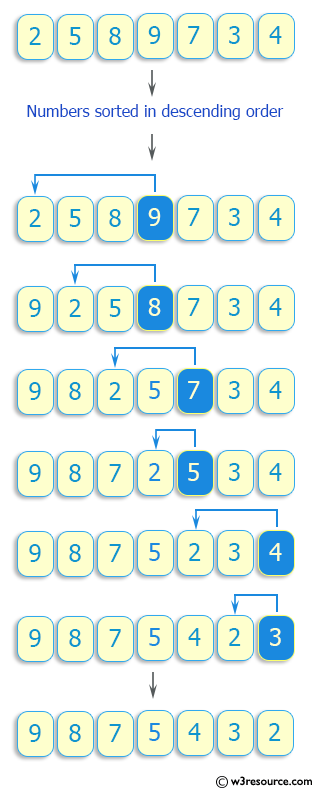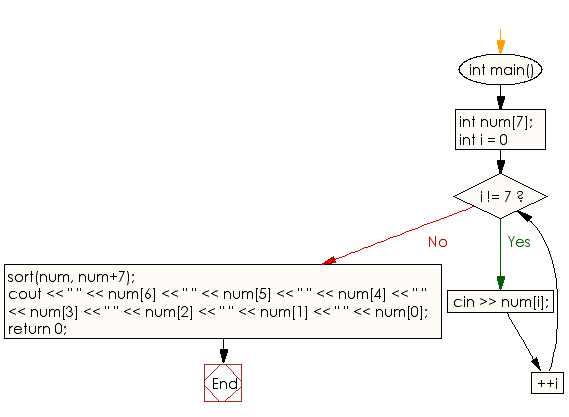﻿ C++ : Read seven numbers and sorts them in descending order

# C++ Exercises: Read seven numbers and sorts them in descending order

## C++ Basic: Exercise-68 with Solution

Write a C++ program that reads seven numbers and sorts them in descending order.

Pictorial Presentation:Sample Solution:

C++ Code :

``````#include <iostream>
using namespace std;

int main() {
int num;
for (int i = 0; i != 7; ++i) {
cin >> num[i];
}
sort(num, num+7);
cout << " " << num  << " " << num  << " " << num  << " " << num  << " " << num << " " << num << " " << num;
return 0;
}
``````

Sample Output:

```Sample Input
2 5 8 9 7 3 4
sample Output
9 8 7 5 4 3 2
```

Flowchart:C++ Code Editor:

What is the difficulty level of this exercise?

﻿

## C++ Programming: Tips of the Day

Why is there no std::stou?

The most pat answer would be that the C library has no corresponding "strtou", and the C++11 string functions are all just thinly veiled wrappers around the C library functions: The std::sto* functions mirror strto*, and the std::to_string functions use sprintf.

Ref: https://bit.ly/3wtz2qA

We are closing our Disqus commenting system for some maintenanace issues. You may write to us at reach[at]yahoo[dot]com or visit us at Facebook